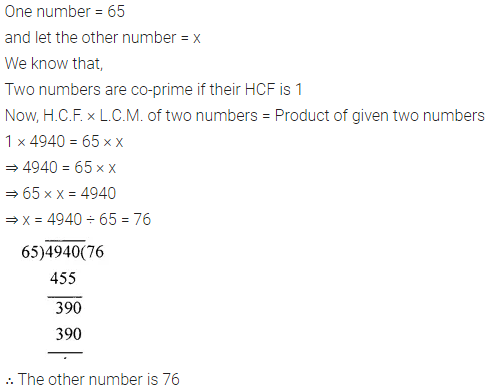ML Aggarwal Class 6 Solutions Chapter 4 Playing with Numbers Check Your Progress for ICSE Understanding Mathematics acts as the best resource during your learning and helps you score well in your exams.

## ML Aggarwal Class 6 Solutions for ICSE Maths Chapter 4 Playing with Numbers Check Your Progress

Question 1.
Write all factors of:
(i) 88
(ii) 105
(iii) 96
Solution:Question 2.
Find the common mutliples of 8 and 12.
Solution: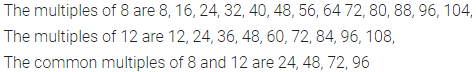Question 3.
Which of the following pairs of numbers are co-prime?
(i) 25 and 105
(ii) 59 and 97
(iii) 161 and 192
Solution: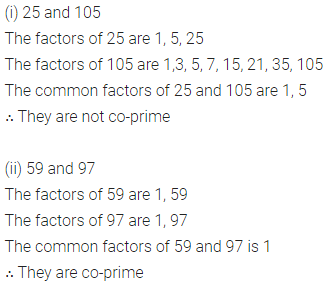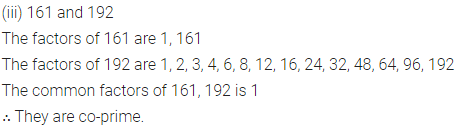Question 4.
Using divisibility tests, determine which of the following numbers are divisible by 4, 6, 8, 9 or 11:
(i) 197244
(ii) 613440
(iii) 4100448
Solution: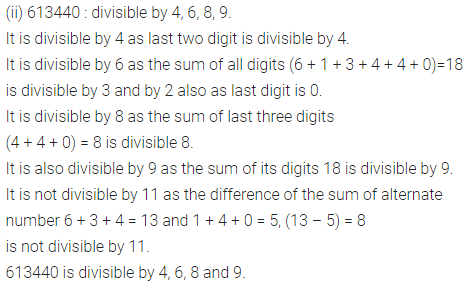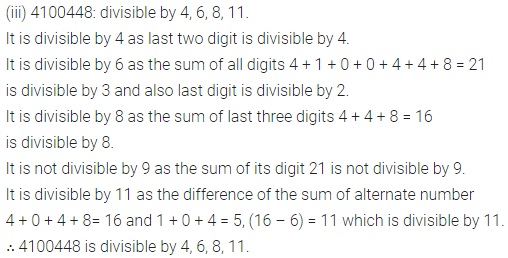Question 5.
In 92 * 389, replace * by a digit so that the number formed is divisible by 11.
Solution:Question 6.
Find the prime factorisation of the following numbers:
(i) 168
(ii) 2304
Solution: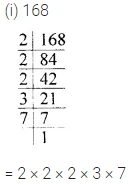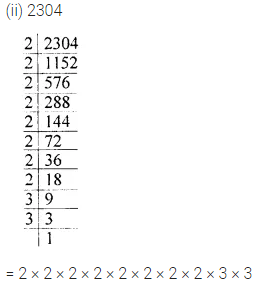Question 7.
Find the G.C.D. of the given numbers by prime factorisation method :
(i) 24,45
(ii) 180, 252, 324
Solution: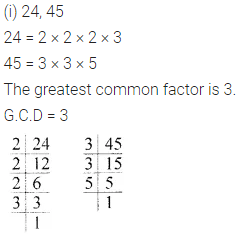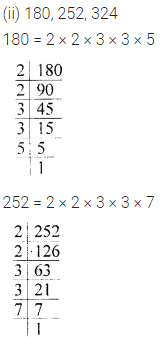Question 8.
Find the H.C.F of the given numbers by division method.
(i) 54, 82
(ii) 84, 120, 156
Solution: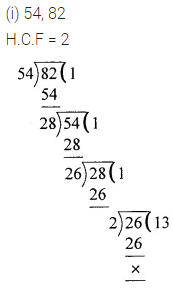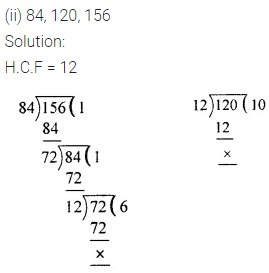Question 9.
Find the L.C.M of the given numbers by prime factorisation method.
(i) 27, 90
(ii) 36, 48, 210
Solution: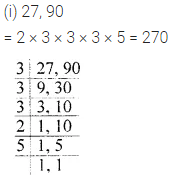Question 10.
Find the L.C.M of the given numbers by division method:
(i) 48, 60
(ii) 112, 168, 266
Solution: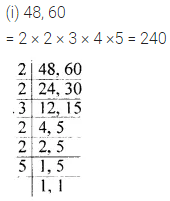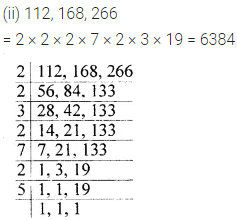Question 11.
Find the greatest number which divides 2706, 7041 and 8250 leaving remainder 6, 21 and 42 respectively.
Solution: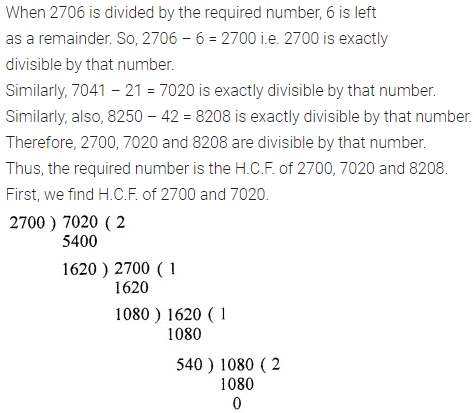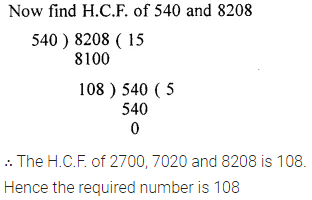Question 12.
Find the least number which on decreasing by 20 is exactly divisible by 18, 21, 28 and 30.
Solution:Question 13.
There are three heaps of rice weighing 120 kg, 144 kg and 204 kg. Find the maximum capacity of a bag so that the rice of each heap can be packed in exact number of bags.
Solution:Question 14.
Three bells are ringing continuously at intervals of 30, 36 and 45 minutes respectively. At what time will they ring together again if they ring simultaneously at 8 a.m.
Solution: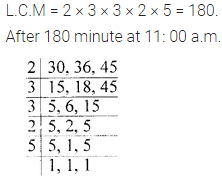Question 15.
Two numbers are co-prime and their L.C.M. is 4940. If one of the numbers is 65, find the other number.
Solution: